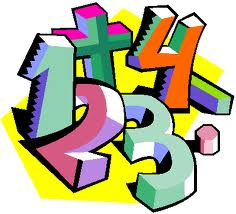## Wednesday, December 24, 2008YOUR UNIQUE DAILY NUMBER

1 2 3 4 5 6 7 8 9

A B C D E F G H I

J K L M N O P Q R

S T U V W X Y Z

The above chart gives you the numerical value for every letter in the alphabet.
Ex: C = 3, R = 9, N = 5

NAME:

_B_I_L_L _________L_E_E___________W_I_L_S_O_N

_2_9_3_3 _________3_5_5_____________5_9_3_1_6_5

(2+9+3+3)_________(3+5+5)___________(5+9+3+1+6+5)

(17)-->(1+7)________(13)-->(1+3)_________(29)-->(2+9)

now 8_______+_________4________+__________11------------> = 23 or
(2+3) = 5 for your total name number.

--------------------------------------------------------------------------------

Remember: reduce all two digits numbers to a signal digit 17(1+7)=8 with ONE
EXCEPTION:

Notice above I didn't reduce the 11.

All double digit numbers --> 11, 22, 33, 44....99 are considered MASTER NUMBERS
and no matter WHERE they are found, they are NEVER, NEVER reduced!

--------------------------------------------------------------------------------

DAILY NUMBER that is yours alone. Very, very doubtful someone will have the
same, exact numbers as yours.